# Triangular Based Pyramid

Here we will learn about triangular based pyramids, including what a triangular based pyramid is and what features it has.

There are also triangular based pyramid worksheets based on Edexcel, AQA and OCR exam questions, along with further guidance on where to go next if you’re still stuck.

## What is a triangular based pyramid?

A triangular based pyramid is a three-dimensional shape with 4 flat triangular faces, 6 edges and 4 vertices. Another name for a triangular based pyramid is tetrahedron.

A pyramid is a polyhedra with a flat base and triangular sides which meet at the apex. The flat base can be any polygon. Pyramids can come in different forms such as a square pyramid, a rectangular pyramid or even a pentagonal pyramid.

### What is a triangular based pyramid?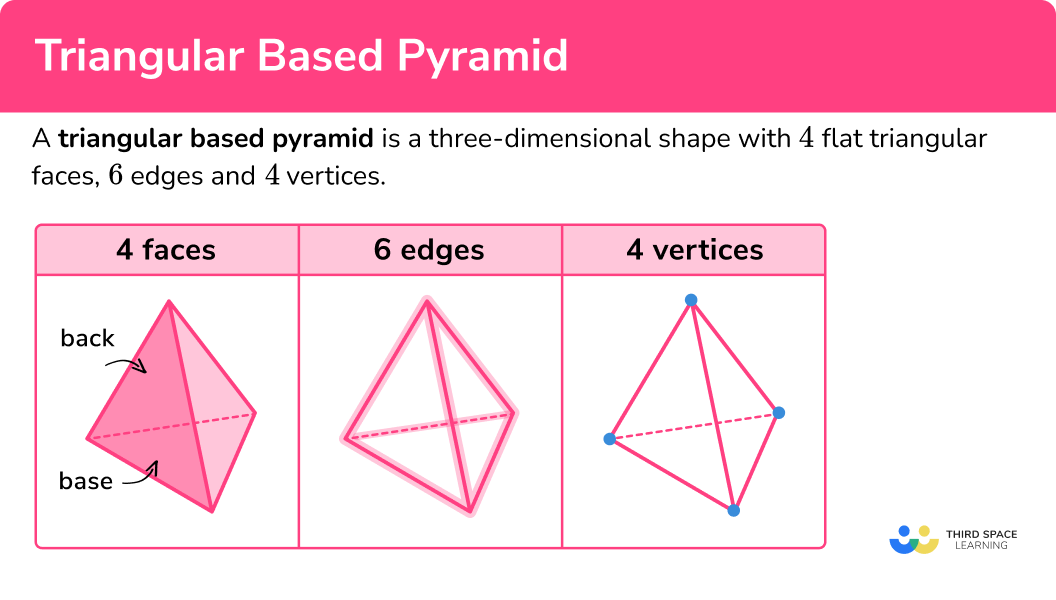### Net of a triangular based pyramid

The net of a triangular based pyramid is made of 4 triangles. If the faces are all equilateral triangles it is known as a regular tetrahedron. The prefix “tetra” means “ 4 ” and comes from the 4 triangular faces.

### Volume of a triangular based pyramid

The volume of a triangular based pyramid can be found by using the formula

\text{Volume}=\cfrac{1}{3}\times \text{area of base} \times \text{height}.

You may be given the area of the triangular base or be asked to work it out. The pyramid height should be perpendicular to its base.

This can be written as

V=\cfrac{1}{3}Bh ,

where

• V represents the volume of the pyramid,
• B represents the area of the base of the pyramid,
• h represents the perpendicular height of the pyramid.

### Surface area of a triangular based pyramid

The surface area of a triangular based pyramid is calculated by finding the area of each of the triangular faces and adding them together.

If the faces of the triangular pyramid are all the same size, we can find the area of one face and multiply it by 4.

If the faces are different, we need to work out the area of each face and add them together.

For example, find the surface area of the triangular based pyramid.

Area of each triangular face = 6 \ cm^2 .

There are 4 triangular faces so multiply 6 \ cm^{2} by 4 to give 24 \ cm^{2}.

Total surface area = 24 \ cm^{2}.

### Right triangular pyramid

A right triangular pyramid is a triangular pyramid which has an equilateral triangle as its base and the apex is directly above the centre of the triangle base, forming a right angle. The other 3 faces are isosceles triangles.

## How to calculate the volume of a triangular based pyramid

In order to calculate the volume of a triangular based pyramid:

1. Use the formula for the volume of a pyramid.
3. Write the answer with the correct units.

### Explain how to calculate the volume of a triangular based pyramid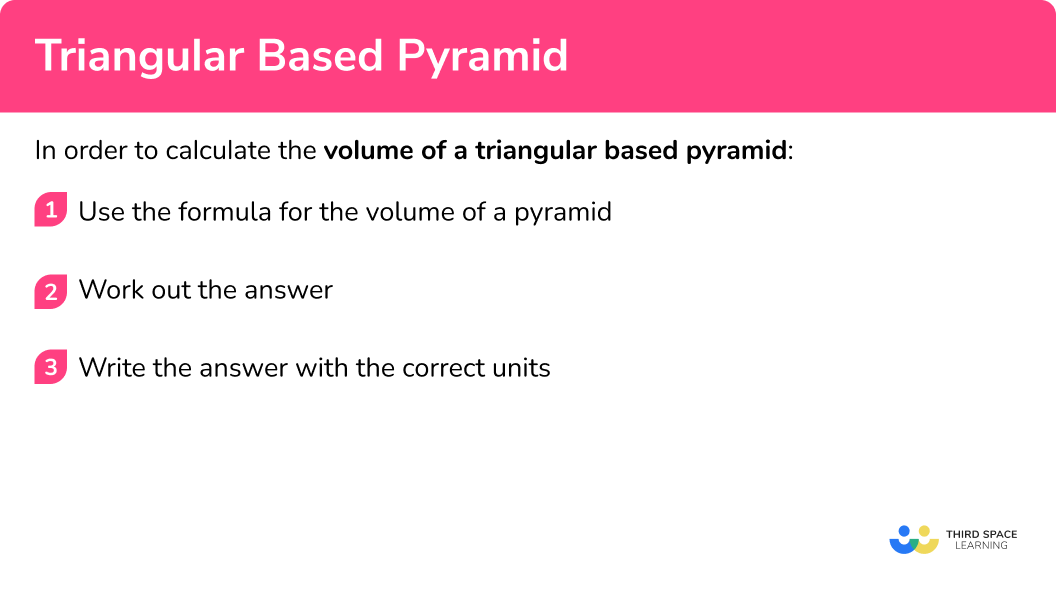## Triangular based pyramid examples

### Example 1: calculating the volume

Find the volume of the pyramid. The area of the base is 20 \ cm^{2}.

1. Use the formula for the volume of a pyramid.

The volume of a triangular pyramid can be found by using the formula

V=\cfrac{1}{3}Bh.

We can substitute the values we have been given into the formula,

V=\cfrac{1}{3}\times 20 \times 6.

V=\cfrac{1}{3}\times 20 \times 6 =40

3Write the answer with the correct units.

The volume is 40 \ cm^{3}.

### Example 2: calculating the volume

Calculate the volume of the triangular pyramid with area of triangular base 30 \ cm^{2} and perpendicular height 12 \ cm.

The volume of a triangular pyramid can be found by using the formula

V=\cfrac{1}{3}Bh.

We can substitute the values we have been given into the formula,

V=\cfrac{1}{3}\times 30 \times 12.

V=\cfrac{1}{3}\times 30 \times 12 =120

The volume is 120 \ cm^{3}.

### Example 3: calculating height given the area

Find the height of the pyramid with volume 150 \ cm^{3} and the base area of 60 \ cm^{2}.

The volume of a triangular pyramid can be found by using the formula

V=\cfrac{1}{3}Bh.

We can substitute the values we have been given into the formula,

150=\cfrac{1}{3}\times 60 \times h.

This can be rearranged to give

h=\cfrac{150\times 3}{60}=7.5.

The height of the pyramid is 7.5 \ cm.

### Example 4: calculating base area given the height of the pyramid

The volume of the pyramid below is 40 \ m^{3}.

Find the base area.

The volume of a triangular pyramid can be found by using the formula

V=\cfrac{1}{3}Bh.

We can substitute the values we have been given into the formula,

40=\cfrac{1}{3}\times B\times 10.

This can be rearranged to give

B=\cfrac{40\times 3}{10}=12.

The area of the base of the pyramid is 12 \ m^{2}.

### Example 5: working out the total surface area

Find the total surface area of a triangular pyramid made of equilateral triangles. The area of each face is 20 \ cm^2 .

This step is not needed, because we are working out the surface area.

A triangular pyramid is made of 4 triangular faces. We are told that all 4 faces are the same. Each face has an area of 20 \ cm^{2}. Therefore the total surface area can be found by multiplying 20 by 4.

4\times 20=80

The total surface area is 80 \ cm^{2}.

### Example 6: working out the total surface area using Pythagoras’ theorem or trigonometry

Find the total surface area of a triangular pyramid made of equilateral triangles. The side length of each edge is 5 \ cm. Leave your answer in its exact form.

This step is not needed, because we are working out the surface area.

A triangular pyramid is made of 4 triangular faces. We are told that all 4 faces are the same. Therefore the total surface area can be found by multiplying the area of one face by 4.

First we need to find the area of an equilateral triangle with side length 5 \ cm.

The area can be found in two ways, one using Pythagoras’ theorem and the second using trigonometry.

Method 1: Using Pythagoras’ theorem. We can find perpendicular height of the triangle.

h=\sqrt{5^2-2.5^2}=\cfrac{5\sqrt{3}}{2}

The area of the triangle is then found,

\text{area}=\cfrac{1}{2}bh=\cfrac{1}{2}\times 5\times \cfrac{5\sqrt{3}}{2}=\cfrac{25\sqrt{3}}{4}.

The total surface area can then be found,

4\times \cfrac{25\sqrt{3}}{4}=25\sqrt{3}.

Note: The height of the triangle could also be found using right-angle trigonometry.

\text{height}=5sin(60)=5\times \cfrac{\sqrt{3}}{2}=\cfrac{5\sqrt{3}}{2}

Note: \sin(60) is one of the important exact trigonometric values to learn.

Method 2: Using further trigonometry. We can find the area of the triangle by using the formula

\text{area}=\cfrac{1}{2}absinC.

a=5, \ b=5 \ \text{and} \ C=60

\text{area}=\cfrac{1}{2}absinC=\cfrac{1}{2}\times 5\times 5\times sin(60)=\cfrac{25\sqrt{3}}{4}

The total surface area can then be found,

4\times \cfrac{25\sqrt{3}}{4}=25\sqrt{3}.

The total surface area is 25\sqrt{3}cm^{2}.

### Common misconceptions

• The height is the perpendicular height

The height of the pyramid needs to be the perpendicular height. This is the height that is at a right-angle to the base.

• Use the correct units

Remember to use cubic units for volume such as cm^3 or m^{3}. Square units are for area, for example cm^2 or m^2.

• Use the correct volume formula

There are many different volume formulas in maths. Make sure that you use the correct one to work out the volume of a pyramid.

\text{Volume}=\cfrac{1}{3}\times \text{area of base} \times \text{height}

Or

V=\cfrac{1}{3}Bh

• Be accurate

When there are two or more steps in your workings, do not round your workings. Only round at the end of your solution so that your answer is accurate.

• Take care with rounding

At the end of the question, make sure you round your answer to the correct number of decimal places or significant figures.

Volume of a pyramid is part of our series of lessons to support revision on pyramids. You may find it helpful to start with the main pyramid lesson for a summary of what to expect, or use the step by step guides below for further detail on individual topics. Other lessons in this series include:

### Practice triangular based pyramid questions

1. Which of these nets are for a triangular based pyramid?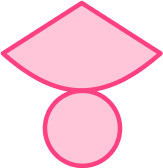A triangular based pyramid has 4 faces. Each of the faces is a triangle.The other nets are a cone, a cube and a square based pyramid.

2. How many edges does a triangular based pyramid have?

3456A triangular based pyramid has 6 edges.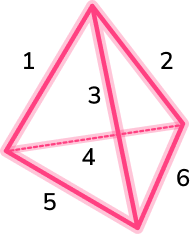3. The area of the base of this pyramid is 40 \ cm^{2}. Find its volume.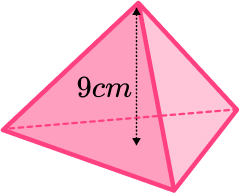360 \ cm^2120 \ cm^2120 \ cm^3360 \ cm^3We can work out the volume of a pyramid by using the formula

V=\cfrac{1}{3}Bh.

We can substitute the values we have been given into the formula and work out the volume,

V=\cfrac{1}{3}\times 40 \times 9=120.

The volume is 120 \ cm^{3}.

4. The volume of a triangular based pyramid is 100 \ cm^{3}. The area of its base is 25 \ cm^{2}. Find the height of the triangular based pyramid.

24 \ cm12 \ cm4 \ cm40 \ cmWe use the formula for volume of a pyramid

V=\cfrac{1}{3}Bh.

We can substitute the values we have been given into the formula,

100=\cfrac{1}{3}\times 25 \times h.

We can rearrange this to find the height,

h=\cfrac{100\times 3}{25}=12.

The height is 12 \ cm.

5. The volume of a triangular based pyramid is 80 \ cm^{3}. The height is 9.7 \ cm. Find the area of the base of the triangular based pyramid. Give your answer correct to 1 decimal place.

24.8 \ cm^28.2 \ cm^268.0 \ cm^224.7 \ cm^2We use the formula for volume of a pyramid,

V=\cfrac{1}{3}Bh.

We can substitute the values we have been given into the formula,

80=\cfrac{1}{3}\times B \times 9.7.

We can rearrange this to find the base area,

B=\cfrac{80\times 3}{9.7}=24.742…

The area of the base is 24.7 \ cm^2 when rounded to 1 decimal place.

6. A triangular based pyramid is made from 4 equilateral triangles. If the area of one of the faces is 21 \ cm^{2}, find the total surface area of the triangular based pyramid.

61 \ cm^384 \ cm^384 \ cm^261 \ cm^2A triangular based pyramid has 4 faces. If all the faces are the same we can find the total surface area by multiplying by 4.4\times 21=84

So the total surface area is 84 \ cm^{2}.

7. Find the total surface area of a triangular pyramid made of equilateral triangles. The side length of each edge is 10 \ cm. Leave your answer in its exact form.

25\sqrt{3} \ cm^250\sqrt{3} \ cm^375\sqrt{3} \ cm^3100\sqrt{3} \ cm^2The area of one face is 25\sqrt{3}. This can be found by using Pythagoras’ theorem.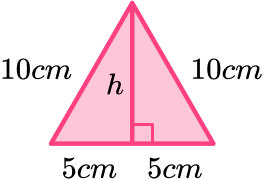h=\sqrt{10^2-5^2}=5\sqrt{3}

\text{area}=\cfrac{1}{2}\times 10\times 5\sqrt{3}=25\sqrt{3}

The area of one face can be multiplied by 4 to find the total surface area.

\text{total surface area}=4\times 25\sqrt{3}=100\sqrt{3}

### Triangular based pyramid GCSE questions

1. The diagram shows a 3D shape.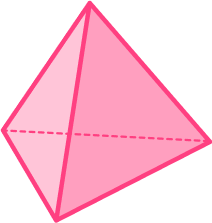Circle the correct name for the 3D shape.

(1 mark)

ATetrahedron

(1)

2. The diagram shows a triangular based pyramid.(a) How many faces does a triangular based pyramid have?

(b) How many vertices does a triangular based pyramid have?

(2 marks)

(a) 4

(1)

(b) 4

(1)

3. The diagram shows a pyramid.

B is directly above A.

AB = 5 \ cm AC = 6 \ cm AD = 4 \ cm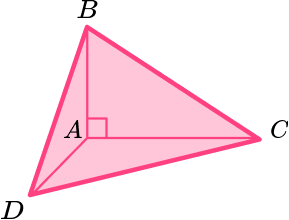Calculate the volume of this pyramid.

(4 marks)

Area of triangular base is \cfrac{1}{2} \times 4 \times 6=12 .

(1)

Volume of pyramid is \cfrac{1}{3} \times 12 \times 5 .

(1)

20

(1)

cm^3

(1)

4. A tetrahedron is made from 4 equal triangles.

The total surface area is 240 \ cm^{2}.

Find the area of one of the faces.

(2 marks)

240\div 4=60

(1)

60 \ cm^2

(1)

5. A tetrahedron is made from 4 equilateral triangles with side length 6 \ cm.

Find the total surface area of the tetrahedron.

(4 marks)

Height of triangle 3\sqrt{3} or sin(60)=\cfrac{\sqrt{3}}{2} .

For using exact value of sin(60) or finding the height of the equilateral triangle.

(1)

Area of one triangle is 9\sqrt{3}.

(1)

4 \times 9\sqrt{3}

(1)

36\sqrt{3} \ cm^2

(1)

## Learning checklist

You have now learned how to:

• Recognise a triangular based pyramid and its features
• Calculate the volume of a triangular based pyramid
• Solve problems involving the volume
• Find the total surface area of a triangular based pyramid

## Still stuck?

Prepare your KS4 students for maths GCSEs success with Third Space Learning. Weekly online one to one GCSE maths revision lessons delivered by expert maths tutors.

Find out more about our GCSE maths tuition programme.